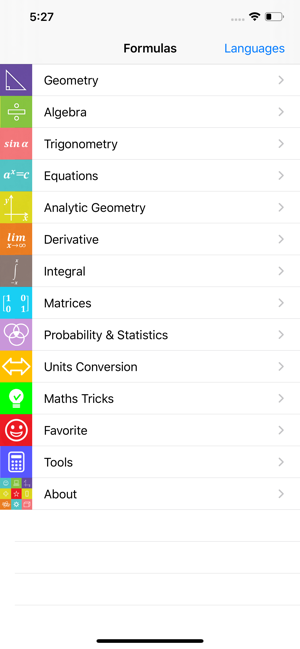# Maths Formulas Free

## Review Overview

### 6.5

#### Maths Formulas Free: An App for Math

Summary : A Resource of Equations and Formulas Waiting to be Used

[asa_item id=”645431389″]

Maths Formulas Free is an index of commonly used equations and formulas for many mathematical disciplines such as Geometry, Algebra, Trigonometry, and more. This app also contains Unit Conversion Tables, Math Tricks, and a Tools option. To access this information, users must select the topic of interest from the menu on the left of the app by tapping it. The app will then present users with subtopics, which helps further specify the topic. As users select the different topics and subtopics, the app shows them detailed information pertaining to their choices, and users are able to scroll through the content. Lastly, if users tap the Tools option, they will be able to access calculators to find the length, area, volume, mass, and speed of objects. The Tools option also lets users find the area of different 2D shapes.

### Instructional Ideas

1. Teachers can present students with a word problem, and before students solve it, they will have to use this app in order to select the correct formula. Next, the teacher can facilitate a class conversation about the formula and this app by asking questions such as: (1) Why do you think this formula will work with this word problem?, (2) How would you insert the data from the word problem into the formula?, and (3) Are there any other formulas you think may work? After the conversation, students can solve the word problem using the formula.
2. Teachers can show students a graph, shape, or figure that has some variables included in its depiction. Next, students will have to identify which equation or formula they would need to use when working with the content presented to them. Finally, students can use the equation or formula to find and add the missing variables to the graph, shape, or figure.
3. Teachers can assign students an equation or formula from this app. Students will then have to draft a word problem that can be solved by using the equation or formula they were assigned. Students can post their word problem and equation or formula to a class website, and teachers can require the other students in the class to solve the problem using the equation or formula.
4. When studying geometry or physics, teachers can have students use the calculators under the
“Tools” option to help them solve problems.
 A1. Rigor A2. 21st Century Skills A3. Conn. to Future Learning A4. Value of Errors A5. Feedback to Teacher A6. Level of Material A7. Cooperative Learning A8. Accom. of Individual Diff.
 B1. Ability to Save Progress B2. Platform Integration B3. Screen Design B4. Ease of Use B5. Navigation B6. Goal Orientation B7. Information Presentation B8. Media Integration B9. Cultural Sensitivity
 C1. Learner Control C2. Interactivity C3. Pace C4. Flexibility C5. Interest C6. Aesthetics C7. Utility

### Screenshots

•••••••